Get instant live expert help with Excel or Google Sheets“My Excelchat expert helped me in less than 20 minutes, saving me what would have been 5 hours of work!”

#### Post your problem and you’ll get expert help in seconds.

Your message must be at least 40 characters
Our professional experts are available now. Your privacy is guaranteed.

# How To Create A Running Total In Excel

Whenever we wish to track the total of a given data set such as the totals sales for each day, we can use the running total. This function will calculate the cumulative sum (running total) in Excel every time new data is added to the existing list. There are many ways to create the running total in Excel, but in this tutorial, we will create the running total using the SUM function and Pivot Table.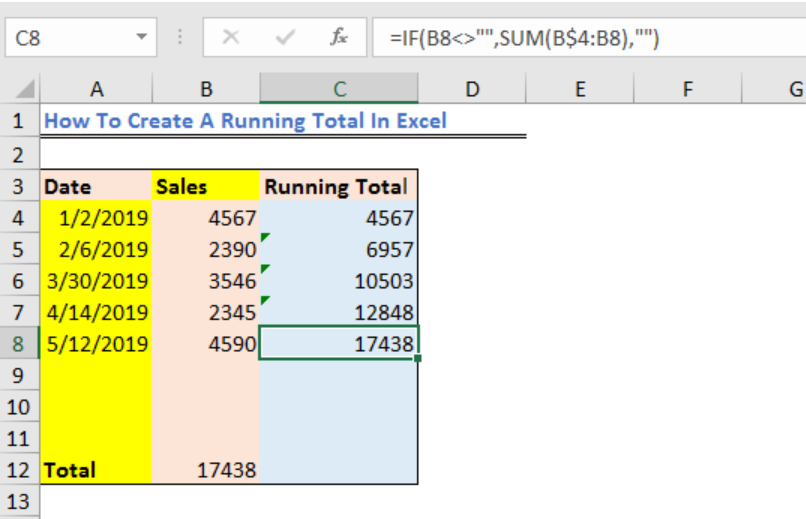Figure 1 – Excel running total

## Running Total with the SUM formula

• We will create a hypothetical data list to be used in calculating the running total using the SUM function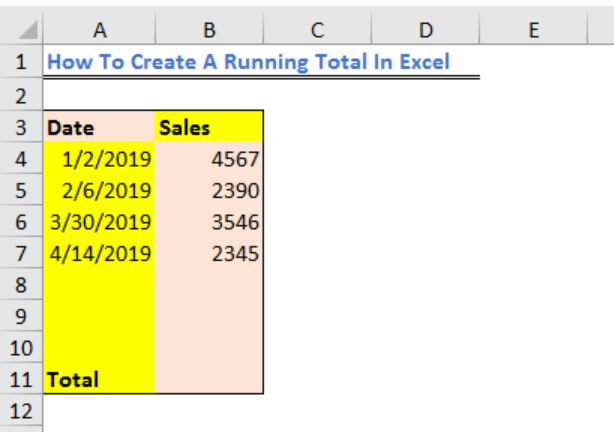Figure 2 – Data for running sum

• In Cell B12, we will enter a simple SUM function to calculate the sum of our data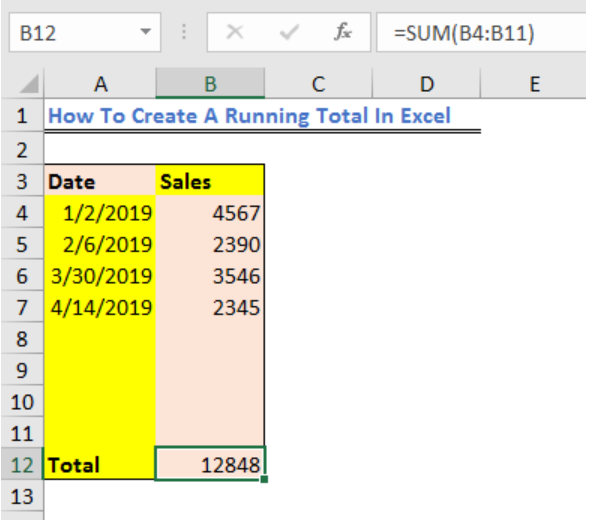Figure 3 – How to create a running total in excel

• In Cell C4, we will enter the SUM function setting the first cell (B\$4) as the mixed reference. This means we will fix the reference to Row 4 by adding a \$ symbol in front of the row number and then set again the Cell B4 as a relative reference cell, as shown below.

`=SUM(B\$4:B4)`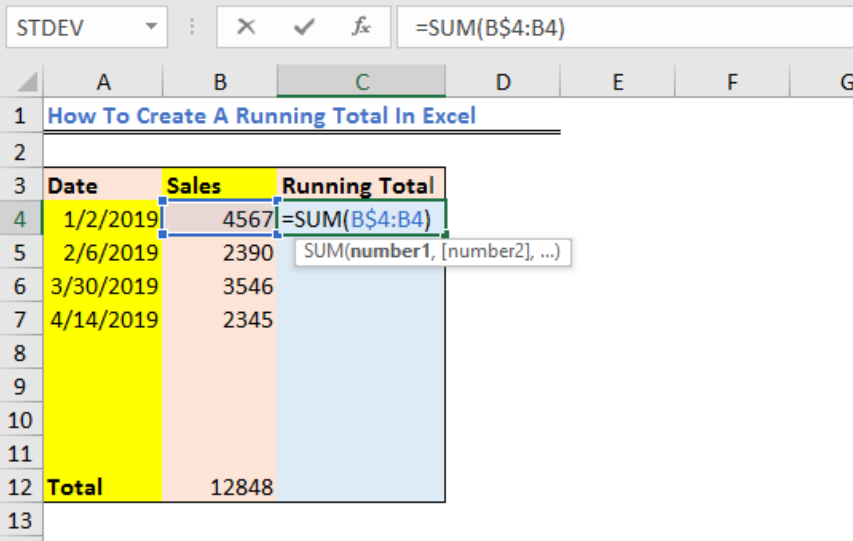Figure 4 – Excel running total

• We will select Cell C4 and using the fill handle tool, drag down the formula into the cells below.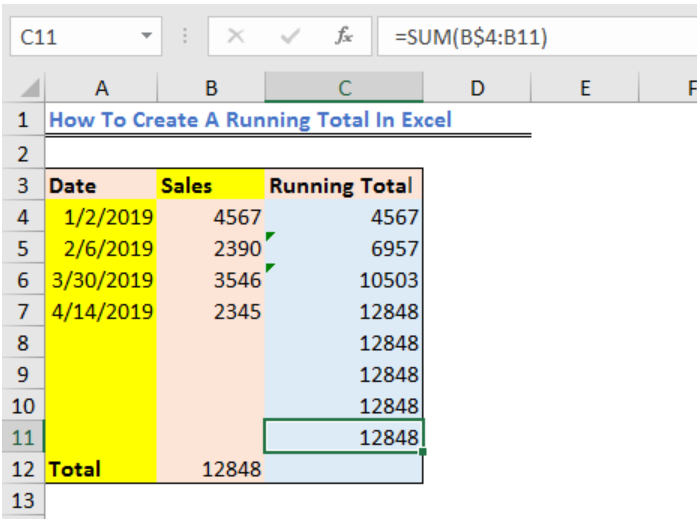Figure 5 – How to keep a running total in excel

• We will go back to Cell C4 and modify the formula with an IF function as shown below. This means that if Cell B4 is not empty, then the If function in Cell C4 should display the cumulative sum, else, display an empty string

`=IF(B4<>””,SUM(B\$4:B4)),””)`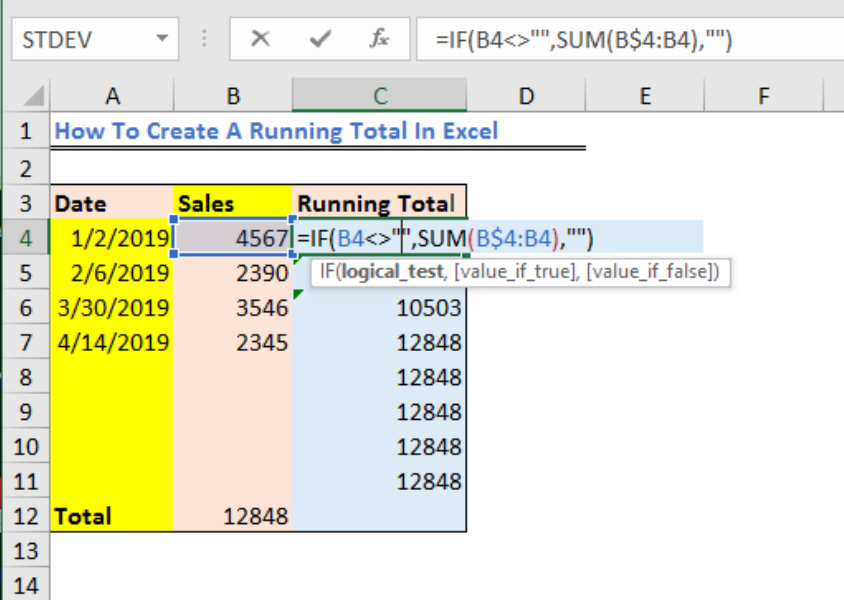Figure 6 – Running total in excel

• Now, we will drag down the formula to modify entries in the other cells within Column C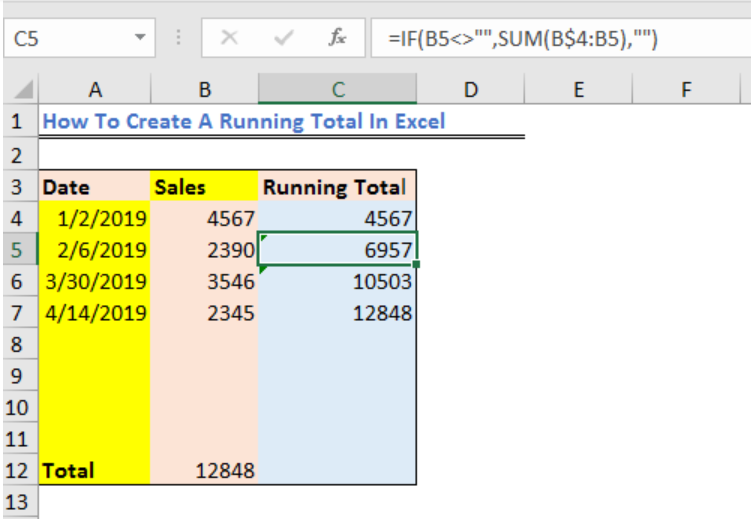Figure 7 – Excel running total

• If we enter a new value in Cell B8, the data in Cell B4 automatically changes to reflect the added data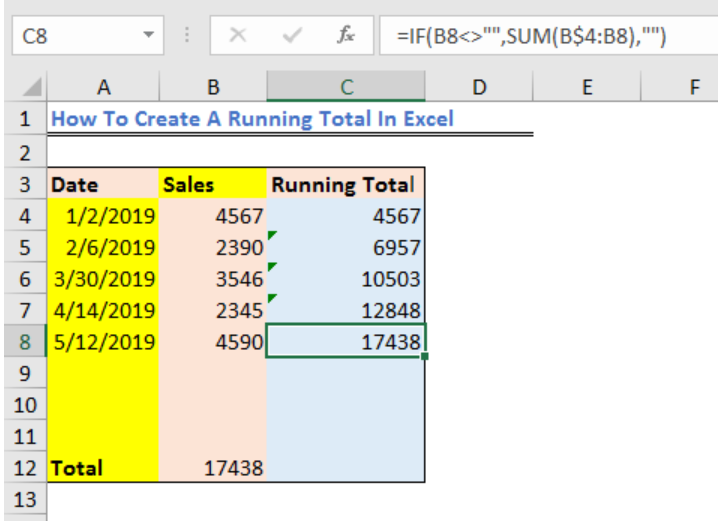Figure 8 – How to keep a running balance in excel

## Running Total with Pivot Table

Step 1 – We will prepare a hypothetical data list as displayed below.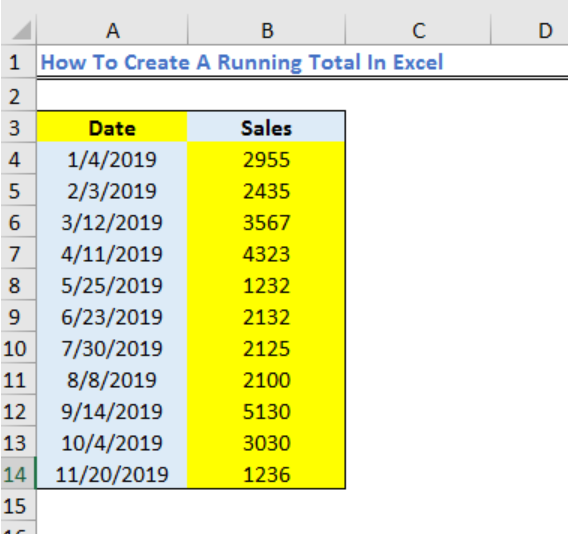Figure 9 – Running total in excel

Step 2 – We will highlight the data range and select Pivot Table from the Insert Tab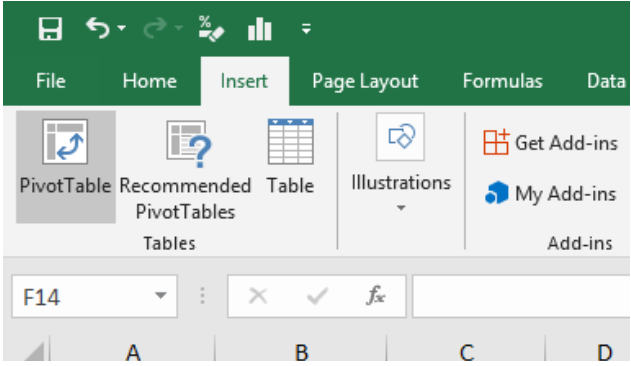Figure 10 – Running total

Step 3 – We will add the Date field to the Rows area of the pivot table and add the sales field to the values area. Again, we will add the sales field to the Row area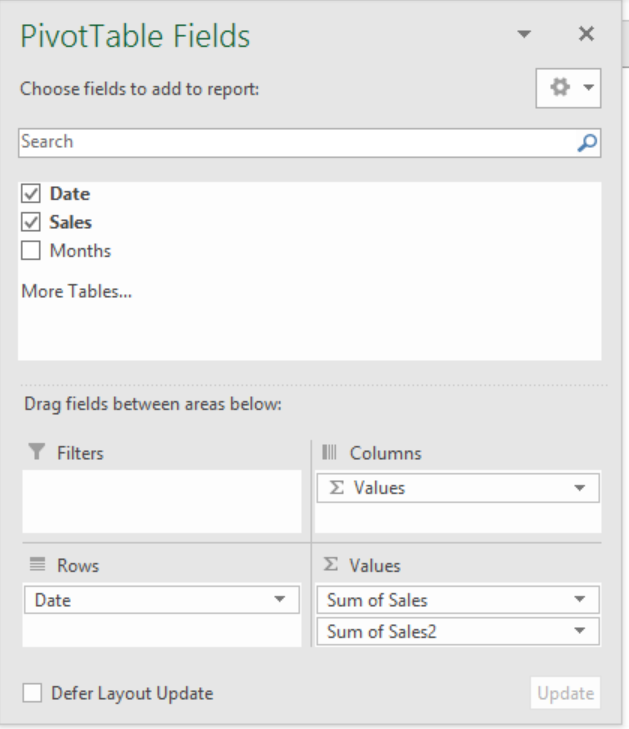Figure 11 – Excel running balance

Step 4 – Now, we will have two identical sales field, of which one of them will be labelled as the Sum of Sales 2.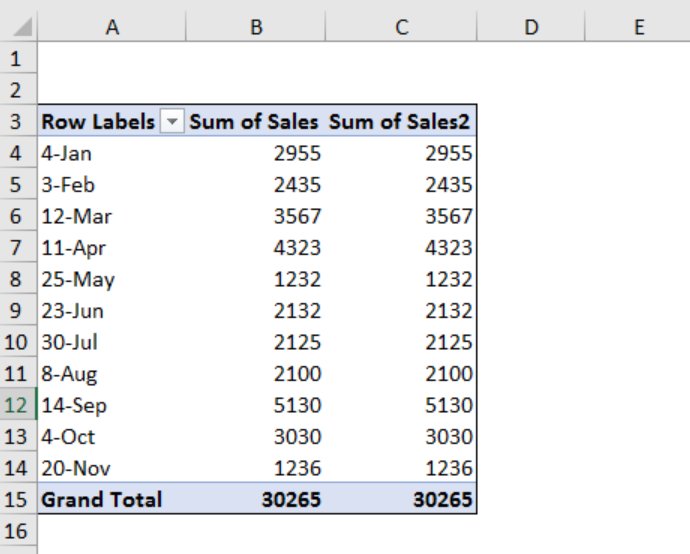Figure 12 – Running total in excel

Step 5 – We will right-click on any value in the Sum of sales field 2 and select Show Value as. We will select Running Total In from the drop-down menu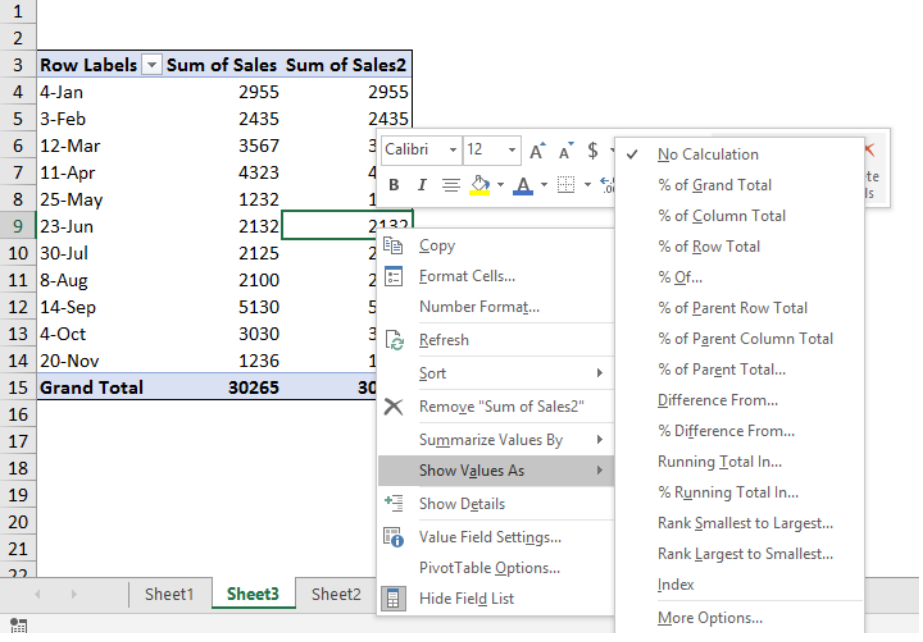Figure 13 – Running balance in Excel

In the Show Value As (Sum of Sales2) window, we will select Date as the base field because we want to show the running total by date.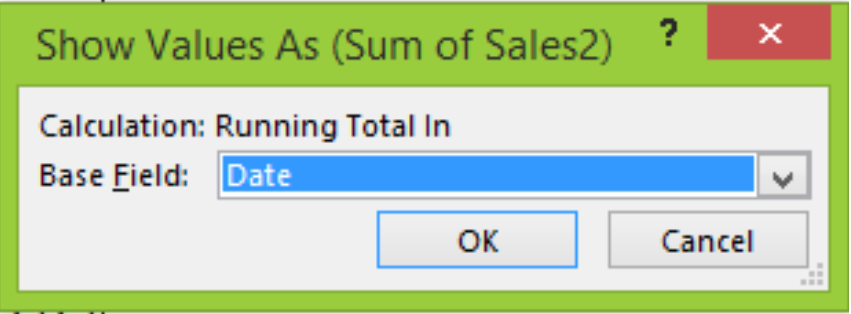Figure 14 – running sum

Therefore, whenever we add or delete a row in the source data, we can refresh the Pivot Table by right-clicking and select Refresh to see the new result.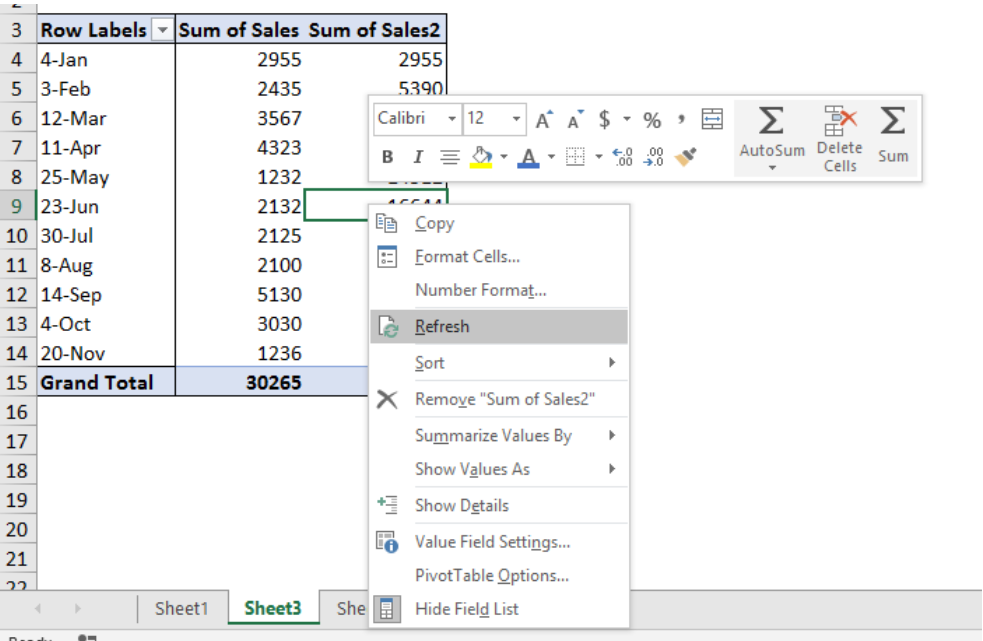Figure 15 – How to create a running total in excel

## Instant Connection to an Expert through our Excelchat Service

Most of the time, the problem you will need to solve will be more complex than a simple application of a formula or function. If you want to save hours of research and frustration, try our live Excelchat service! Our Excel Experts are available 24/7 to answer any Excel question you may have. We guarantee a connection within 30 seconds and a customized solution within 20 minutes.